Next: Smoothness and monotonicity constraints Up: .  Introduction: Variable gain image Previous: Estimation in the presence

## Estimating camera response function

From (4), we may find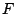by minimizing the sum of squared errors: =_x _y ( F(f_1(x)) - F(f_2( x )) + K ) ^2

Sincecan only be found up to a single unknown scalar constant,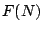is fixed at zero3, whereis the number of greyvalues (typically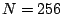).

Equation 4 gives a set of equalities of the form: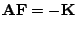, where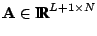, and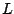is the number of pixels in one of the images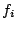. The firstrows of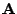are constructed by inserting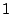in the column index corresponding to the pixel value of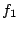and inserting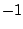into the column index corresponding to the pixel value of: A(x+wy,f_1(x,y))&=&1
A(x+wy,f_2(x,y))&=&-1 where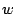is the width of one of the images, and the last row ofis all zeros except its last entry which is 1: A(L+1,N)=1 All unspecified entries of matrixare zero. Vector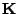is constructed by placing the value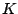in the firstentries and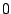in the last entry. This is an overdetermined system of equations.

The solution that minimizes the error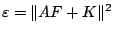in (9) is the maximum likelihood solution according to the noise model of (5), assuming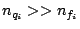, and is given by: ddF = 2A^TAF + 2A^TK = 0 giving F=(A^TA)^-1A^T (-K), assuming additive white Gaussian noise. This solution gives us a way of estimating the camera response function from two or more differently exposed pictures of overlapping subject matter.

Although this system is massively overdetermined, the constraints follow a comparametric form that admits solutions having sinusoidal components (e.g. solutions tend to be wavy'') . (Indeed, comparametric forms are very similar to difference equations as can be seen from the form of (4) which constrains the function over an interval step size of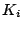allowing ripples in the solution.)

SubsectionsNext: Smoothness and monotonicity constraints Up: .  Introduction: Variable gain image Previous: Estimation in the presence
Steve Mann 2002-05-25